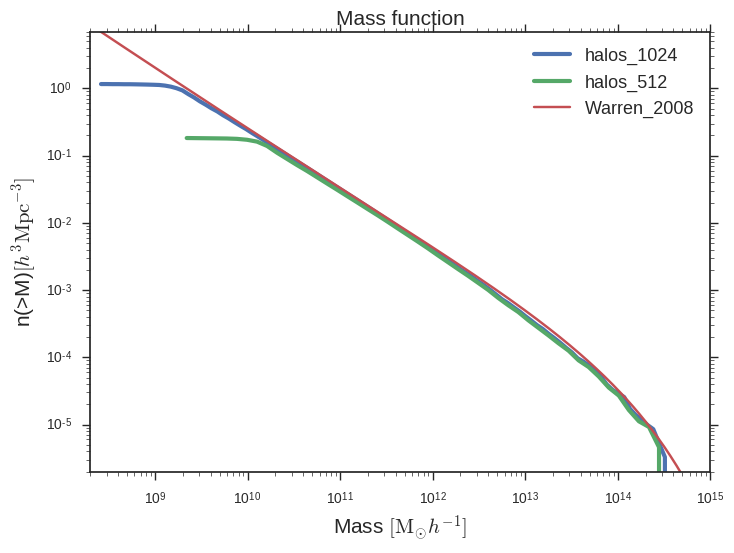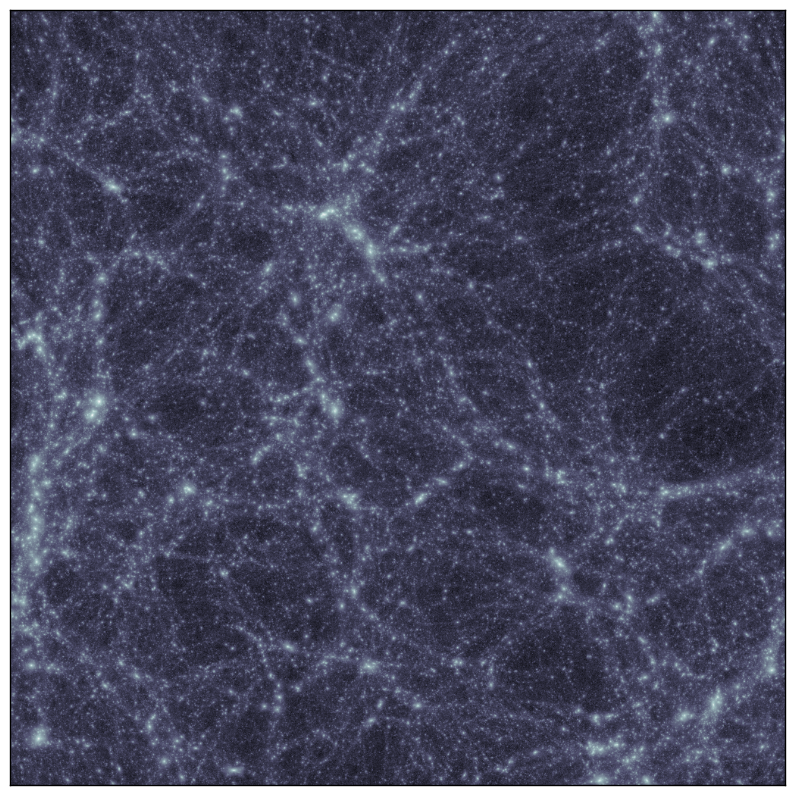SIMULATIONS PARAMETERS:

Box size: 115 Mpc/h

Simulations Details:

Num Particles Particle Mass [M_sun/h] Softening Length [kpc/h]
$128^3$ $6.21 \times 10^{10}$ 19.6
$256^3$ $7.77 \times 10^{9}$ 9.8
$512^3$ $9.71 \times 10^{8}$ 4.9
$1024^3$ $1.21 \times 10^{8}$ 2.4
$2048^3$ $1.52 \times 10^{7}$ 1.2

Cosmology from Plank (2016)Here

Cosmology:

• Omega_m = 0.3089

• Omega_L = 0.6911

• Omega_b = 0.0486

• H0 = 67.74

• sigma_8 = 0.8159

• nspec = 0.967

• transfer = eisenstein

Initial Conditions:

• Z = 100

• Power spectrum from Eisenstein & Hu transfer function

Mass function at z=0 using ROCKSTAR halo catalogs for halo masses:Density projection using Brant’s code (image-snapshot):UPDATE

File with data for plotting mass functions from above Here

Script for plotting mass functions from data file Here

Data file: density projection Here

Data file: potential Here